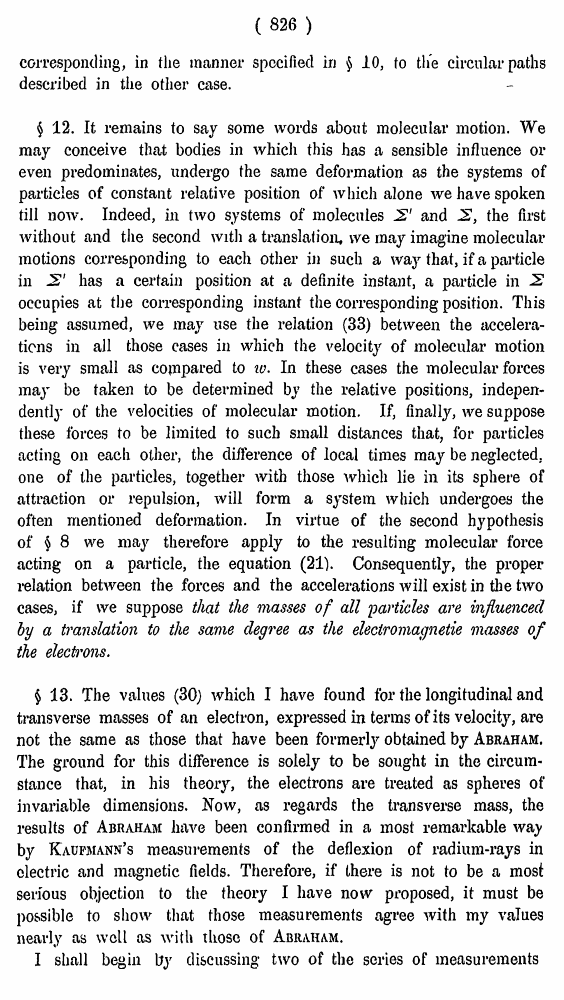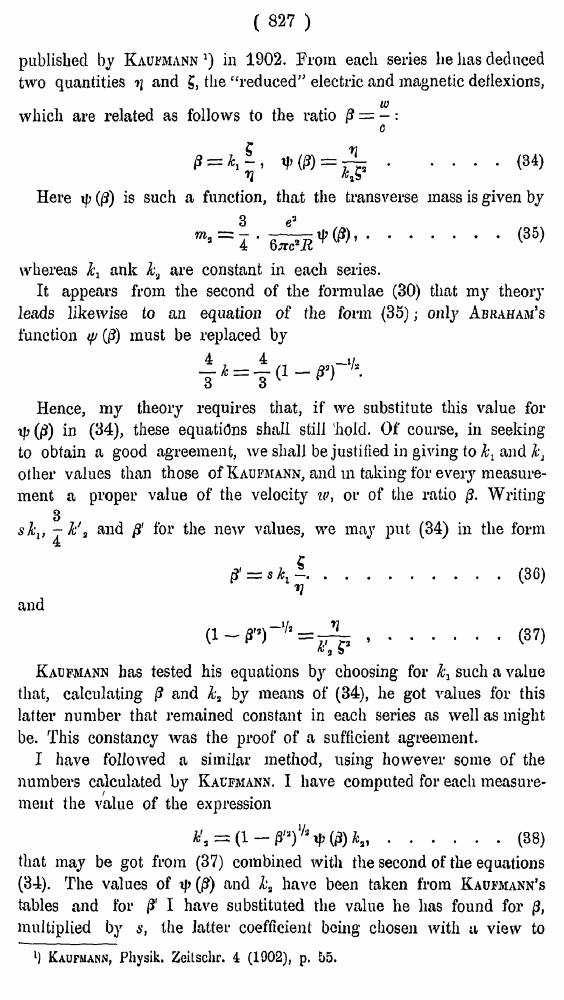# H. A. Lorentz. Electromagnetic phenomena in a system moving with any velocity smaller than that of light. // Proceedings Royal Acad., Amsterdam. Vol. VI., 1904

В начало   Другие форматы   <<<     Страница 826   >>>809  810  811  812  813  814  815  816  817  818  819  820  821  822  823  824  825  826 827  828  829  830  831

( 826 )

corresponding, in the manner specified in § 10, to the circular paths described in the other case.

§ 12. It remains to say some words about molecular motion. We may conceive that bodies in which this has a sensible influence or even predominates, undergo the same deformation as the systems of particles of constant relative position of which alone we have spoken till now. Indeed, in two systems of molecules 2' and 2, the first without and the second with a translation, we may imagine molecular motions corresponding to each other in such a way that, if a particle in 2' has a certain position at a definite instant, a particle in 2 occupies at the corresponding instant the corresponding position. This being assumed, we may use the relation (33) between the accelerations in all those cases in which the velocity of molecular motion is very small as copipared to to. In these cases the molecular forces may be taken to be determined by the relative positions, independently of the velocities of molecular motion. If, finally, we suppose these forces to be limited to such small distances that, for particles acting on each other, the difference of local times may be neglected, one of the particles, together with those which lie in its sphere of attraction or repulsion, will form a system which undergoes the often mentioned deformation. In virtue of the second hypothesis of § 8 we may therefore apply to the resulting molecular force acting on a particle, the equation (21). Consequently, the proper relation between the forces and the accelerations will exist in the two cases, if we suppose that the masses of all particles are influenced by a translation to the same degree as the eleclromagnetie masses of the electrons.

§ 13. The values (30) which I have found for the longitudinal and transverse masses of an electron, expressed in terms of its velocity, are not the same as those that have been formerly obtained by Abraham. The ground for this difference is solely to be sought in the circumstance that, in his theory, the electrons are treated as spheres of invariable dimensions. Now, as regards the transverse mass, the results of Abraham have been confirmed in a most remarkable way by Kaufmann’s measurements of the deflexion of radium-rays in electric and magnetic fields. Therefore, if there is not to be a most serious objection to the theory I have now proposed, it must be possible to show that those measurements agree with my values nearly as well as with those of Abraham.

I shall begin by discussing two of the series of measurements

( 827 )

published by Kaufmann1) in 1902. From each series he has deduced two quantities ■>] and g, the “reduced” electric and magnetic deflexions,

which are related as follows to the ratio

.... (34)

Here (0) is such a function, that the transverse mass is given by

• (35)

whereas k^ ank k3 are constant in each series.

It appears from the second of the formulae (30) that my theory leads likewise to an equation of the form (35); only Abkaham’s function tp (/?) must be replaced by

Hence, my theory requires that, if we substitute this value for tf>(0) in (34), these equations shall still 'hold. Of course, in seeking to obtain a good agreement, we shall be justified in giving to l\ and ks other values than those of Kaufmann, and ui taking for every measurement a proper value of the velocity iv, or of the ratio Writing 3

skv — k\ and ft for tb« "aw vjiIhas we may put (34) in the form

and

#### .......(37)

Kaufmann has tested his equations by choosing for k^ such a value that, calculating |? and kt by means of (34), he got values for this latter number that remained constant in each series as well as might be. This constancy was the proof of a sufficient agreement.

I have followed a similar method, using however some of the numbers calculated by Kacfmann. I have computed for each measurement the value of the expression

......(38)

that may be got from (37) combined with the second of the equations (34). The values of {\$) and k3 have been taken from Kaufmann’s tables and for /J' I have substituted the value he has found for 0, multiplied by s, the latter coefficient being chosen with u view to

l) Kaufmann, Physik. Zeitschr. 4 (1002), p. 55.